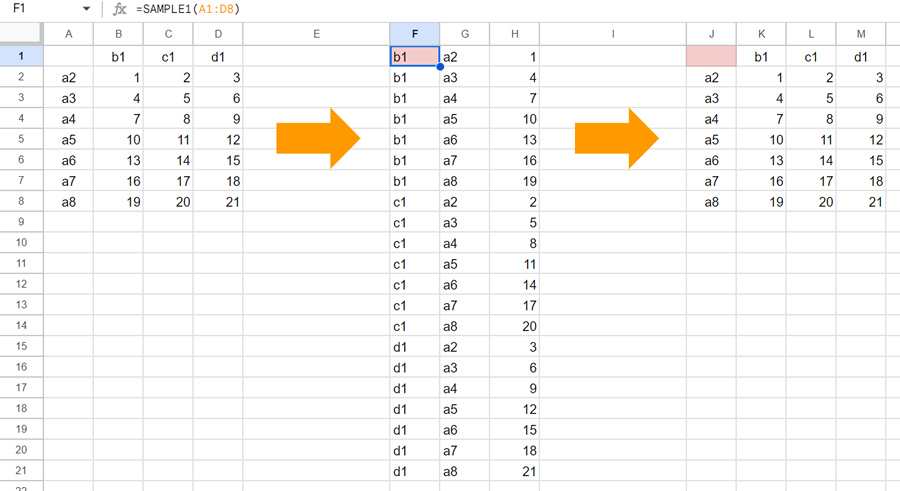GistsThis is a sample script for converting the values on Google Spreadsheet as unpivot (reverse pivot) using Google Apps Script.

## Sample script 1:

const SAMPLE1 = ([[, ...header], ...values]) =>
header.flatMap((h, i) => values.map((t) => [h, t, t[i + 1]]));

• In the sample, the source data is “A1:D8”. And, a custom function of =SAMPLE1(A1:D8) is put into “F1”.
• When this script is used, the result showing the above image (most left table to middle table) is obtained.

## Sample script 2:

const SAMPLE2 = v => {
const [a, b, c] = v.map((_, c) => v.map(r => r[c]));
const ch = [...new Set(a)];
const rh = [...new Set(b)];
const size = rh.length;
const values = [...Array(Math.ceil(c.length / size))].map(_ => c.splice(0, size));
const temp = [[null, ...rh], ...values.map((vv, i) => [ch[i], ...vv])];
return temp.map((_, c) => temp.map(r => r[c]));
}

• In the sample, the source data is “F1:H21”. And, a custom function of =SAMPLE2(F1:H21) is put into “J1”.
• When this script is used, the result showing the above image (middle table to most right table) is obtained.